## Defining Financial Leverage

Financial leverage is a tactic to multiply gains and losses, calculated by a debt-to-equity ratio.

### Learning Objectives

Calculate financial leverage, and recognize the core relationship between risk and return

### Key Takeaways

#### Key Points

• Leverage is a financial tactic to multiply gains and losses, accomplished through borrowing capital on existing assets.
• Through achieving leverage, organizations can grow exponentially faster due to access to far more resources than their assets would generally allow.
• From a financial point of view, financial leverage is calculated as total debt /shareholder equity.
• When assessing financial leverage, it’s important to recognize the implications on the cost of capital. This is to say, all borrowed money comes with interest, and the weighted average cost of capital (WACC) is a critical in understanding the risk.
• The Lehman Brothers were leverage over 30 times when the financial collapse of 2007-2009 occurred. This degree of risk in unsustainable and highly dangerous not only to the organization, but to the economy at large.

#### Key Terms

• leverage: The overall debt divided by shareholder equity. This borrowing allows for the multiplication of gains and losses.

### Why Organizations Leverage Finances

At its simplest, leverage is a tactic geared at multiplying gains and losses. Leveraging existing assets to get exponentially more return can be a risk intensive process, and represents a significant aspect of financial strategy and capital structure. Achieving leverage can enable significant competitive advantages despite the risk, however, as it can accelerate the speed of revenue acquisition exponentially.

The standard way to accomplish leverage is through borrowing, via debt and equity, to invest at a much higher scale than one’s current assets would allow. In order to borrow substantial amounts of capital, firms must pursue a variety of financial sourcing and be able to back up their debts with valuable assets ( collateral ). Even with a great deal of collateral, borrowing big means risking big. Interest rates ensure that the strategic discussions around expanding leverage take into account the risk and return trade offs.

### Measuring Leverage

In finance, the best definitions come in equation format. The standard definition of financial leverage is as follows:

$\mathrm {Financial\;leverage} ={\frac {\mathrm {Total\;Debt} }{\mathrm {Shareholders'\;Equity} }}$

In short, the ratio between debt and equity is a strong sign of leverage. As you may already know, equity is ownership of the organization and pays out fairly significant dividends. Debt is often lower cost access to capital, as debt is paid out before equity in the event of a bankruptcy (thus debt is intrinsically lower risk for the investor).

### Leverage and Capital Costs

The debt to equity ratio plays a role in the working average cost of capital (WACC) as well, as the overall interest on financing represents the break-even point that must be obtained to achieve profitability in a given venture. WACC is essentially the overall average interest an organization owes on the capital it has borrowed for leverage.

Let’s say equity represents 60% of borrowed capital and debt is 40%. This results in a financial leverage calculation of 40/60, or 0.6667. The organization owes 10% on all equity and 5% on all debt. That means that the weighted average cost of capital is (.4)(5) + (.6)(10) – or 8%. For every $10,000 borrowed, this organization will owe$800 in interest. Profit must be higher than 8% on the project to offset the cost of interest and justify this leverage.

### Leverage and the 2007-2009 Recession

A word of caution: Leverage is exponentially more risky the more it is utilized. A useful way to view leverage is the overall existing assets of an organization compared to the amount of money they owe. For example, say you own a company with an overall net worth of $1 million. If you were to be leveraged at a total of 1.5 times (i.e. financed at$1.5 million), this would put you at some risk. Arguably a reasonable amount.

Before Lehman Brothers went bankrupt, they were leveraged at over 30 times ($691 billion in financial leverage compared to$22 billion in assets). A mistake of this scale (on both the lenders and the Lehman Brothers) threatened to topple the global economy itself. It’s important to keep responsibility, accountability, and risk in mind when considering leverage options.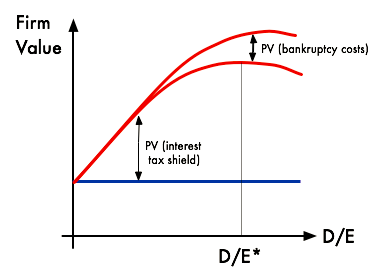Financial Leverage Firm Value Implications: This graph illustrates a theoretical firm value maximizing curve when it comes to a debt-to-equity ratio. All this means is that each organization has the ideal balance between debt and equity, and finding the ‘sweet spot’ is a useful strategic aspect of financial leverage decisions.

## Impacts of Financial Leverage

The use of financial leverage can positively – or negatively – impact a company’s return on equity as a consequence of the increased level of risk.

### Learning Objectives

Describe how leverage can impact a business

### Key Takeaways

#### Key Points

• If value is added from financial leveraging then the associated risk will not have a negative effect.
• At an ideal level of financial leverage, a company’s return on equity increases because the use of leverage increases stock volatility, increasing its level of risk which in turn increases returns.
• If earnings before interest and taxes are greater than the cost of financial leverage than the increased risk of leverage will be worthwhile.

#### Key Terms

• liquidity: Availability of cash over short term: ability to service short-term debt.
• solvency: The state of having enough funds or liquid assets to pay all of one’s debts; the state of being solvent.

### Impacts of Financial Leverage

Taking on debt, as an individual or a company, will always bring about a heightened level of risk due to the fact that income must be used to pay back the debt even if earnings or cash flows go down. From a company’s perspective, the use of financial leverage can positively – or sometimes negatively – impact its return on equity as a consequence of the increased level of risk.

### Impact on Return on Equity

Return on equity is the rate of return on the shareholders ‘ equity of a company’s common stock owners. It measures a firm’s efficiency at generating profits from every unit of shareholders’ equity. Return on equity shows how well a company uses investment funds to generate earnings growth. It can be calculated using the following equation: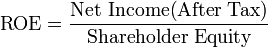Return On Equity: The equation used to calculate return on equity.

At an ideal level of financial leverage, a company’s return on equity increases because the use of leverage increases stock volatility, increasing its level of risk which in turn increases returns. However, if a company is financially over-leveraged a decrease in return on equity could occur. Financial over-leveraging means incurring a huge debt by borrowing funds at a lower rate of interest and using the excess funds in high risk investments. If the risk of the investment outweighs the expected return, the value of a company’s equity could decrease as stockholders believe it to be too risky.

### Leverage, Risk, and Misconceptions

The most obvious risk of leverage is that it multiplies losses. Due to financial leverage’s effect on solvency, a company that borrows too much money might face bankruptcy during a business downturn, while a less-levered company may avoid bankruptcy due to higher liquidity. There is a popular prejudice against leverage rooted in the observation of people who borrow a lot of money for personal consumption – for example, heavy use of credit cards. However, in finance the general practice is to borrow money to buy an asset with a higher return than the interest on the debt. Instead of spending money it doesn’t have, a company actually creates value. On the other hand, when debt is taken on for personal use there is no value being created, i.e., no leveraging.

There is also a misconception that companies enter a higher level of financial leverage out of desperation, referred to as involuntary leverage. While involuntary leverage is certainly not a good thing, it is typically caused by eroding equity value as opposed to the addition of more debt. Therefore, it is typically a symptom of the problem, not the cause.

When evaluating the riskiness of leverage it is also important to factor in the value of the company itself and its activities. If a company borrows money to modernize, add to its product line, or expand internationally, the additional diversification will likely offset the additional risk from leverage. The upshot is, if value is expected to be added from the use of financial leverage, the added risk should not have a negative effect on a company or its investments.

## Combining Operating Leverage and Financial Leverage

To calculate total leverage, we multiply Degree of Operating Leverage by Degree of Financial Leverage.

### Learning Objectives

Calculate a company’s total leverage

### Key Takeaways

#### Key Points

• Total leverage measures the sensitivity of earnings to changes in the level of a company’s sales.
• If the percentage change in earnings and the percentage change in sales are both known, a company can simply divide the percentage change in earnings by the percentage change in sales to determine total leverage.
• Companies usually choose one form of leverage over the other when analyzing potential investments. A company utilizing both forms of leverage undertakes a very high level of risk.

#### Key Terms

• Earnings Per Share: The amount of earnings per each outstanding share of a company’s stock.

### Combining Operating and Financial Leverage

Operating and financial leverage can be combined into an overall measure called “total leverage. ” Total leverage can be used to measure the total risk of a company and can be defined as the percentage change in stockholder earnings for a given change in sales. In other words, total leverage measures the sensitivity of earnings to changes in the level of a company’s sales.

### Methods For Finding Total Leverage

Total leverage can be determined by a couple of different methods. If the percentage change in earnings and the percentage change in sales are both known, a company can simply divide the percentage change in earnings by the percentage change in sales. Earnings can be measured in terms of EBIT, earnings before interest and taxes, or EPS, earnings per share. While EBIT can be determined by referencing a company’s income statement, we can determine earnings per share by dividing the company’s net income by it’s average price of common shares.

Another way to determine total leverage is by multiplying the Degree of Operating Leverage and the Degree of Financial Leverage.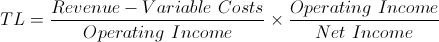Total Leverage Equation 1: Total leverage = DOL x DOF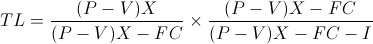Total Leverage Equation 2: Total leverage = DOL x DOF

Therefore: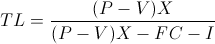Total Leverage Equation 3: TL = Total Leverage. P = Unit Revenue. V = Unit Variable Cost. X = Units Sold. FC = Fixed Costs. I = Interest Expense.

Fully derived, we see that to multiply Degree of Operating Leverage and Degree of Financial Leverage, we subtract fixed costs and interest expense from the total contribution margin (revenue minus variable cost times the number of units sold), and divide total contribution margin by this result. Companies usually choose one form of leverage over the other when analyzing potential investments. One that utilizes both forms of leverage undertakes a very high level of risk.